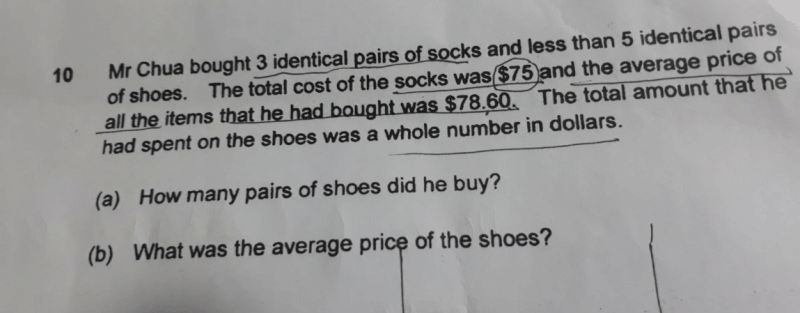# Questiona) Amount spent on socks: \$75
Number of items: Between 4 – 8 (3 socks and between 1 – 5 shoes)

Based on the fact that (Amount spent on shoes = Total amount spent – amount spent on socks)

We know that amount spent on shoes needs to be a whole number, and the amount spent on socks is already a whole number (\$75). Therefore, the Total amount spent must also be a whole number (Whole number – whole number = whole number).

Total amount spent = Average cost of items x total number of items

The average cost of items is \$78.60 so we need to find a number less than 8 that when multiplied by \$78.60, will give a whole number as the product. The only possible number is 5, so Mr Chua bought 5 items in total. 5 items minus the three socks means he bought 2 pairs of shoes.

b) Total amount spent = \$78.60 x 5
= \$393

Amount spent on shoes = \$393 – \$75
= \$318

Average price of each pair of shoes = \$318 ÷ 2
= \$159